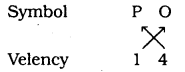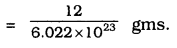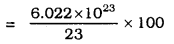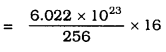# KSEEB Solutions for Class 9 Science Chapter 3 Atoms and Molecules

Students can Download Kannada Chapter 3 Atoms and Molecules Questions and Answers, Summary, Notes Pdf, Siri KSEEB Solutions for Class 9 Science, Karnataka State Board Solutions help you to revise complete Syllabus and score more marks in your examinations.

## Karnataka Board Class 9 Science Chapter 3 Atoms and Molecules

### KSEEB Solutions for Class 9 Science Chapter 3 Intext Questions

Question 1.
In a reaction, 5.3 g of sodium carbonate reacted with 6 g of ethanoic acid. The products were 2.2 g of carbon dioxide, 0.9 g water and 8.2 g of sodium ethanoate. Show that these observations are in agreement with the law of conservation of mass.
Sodium carbonate + ethanoic acid ➝ sodium ethanoate + carbon dioxide + water
Sodium carbonate + ethanoic acid ➝ sodium ethanoate + carbon dioxide + water
5.3 + 6 ➝ 8.2 + 2.2 + 0.9
= 11.3 g = 11.3 g
Weight of reactants is equal to weight of products. This observation is in agreement with the law of conservation of mass.

Question 2.
Hydrogen and Oxygen combine in the ratio or 1 : 8 by mass to form water. What mass of oxygen gas would be required to react completely with 3 g of hydrogen gas?
24 g of oxygen gas would be required to react completely with 3 g of hydrogen gas. Because in water the ratio of the mass of hydrogen to the mass of oxygen is always 1 : 8.

Question 3.
Which postulate of Dalton’s atomic theory is the result of the law of conservation of mass?
The relative number and kinds of atoms are constant in a given compound. This postulate is the result of the law of conservation of mass.

Question 4.
Which postulate of Dalton’s atomic theory can explain the law of definite proportions?
Atoms combine in the ratio of small whole numbers to form compounds. This postulate explains the law of definite proportions.

Question 1.
Define the atomic mass unit.
One atomic mass unit is a mass unit equal to exactly one-twelfth (1/12th) the mass of one atom of carbon-12.

Question 2.
Why is it not possible to see an atom with naked eyes?
Atoms are very small, they are smaller than anything that we can imagine or compare with. Therefore it is not possible to see an atom with naked eyes.

Question 1.
Write down the formulae of:

1. sodium oxide
2. alluminium chloride
3. sodium sulphide
4. magnesium hydroxide

1. sodium oxide: Na2O
2. aluminium chloride: Al2Cl3
3. sodium sulphide : NaS
4. magnesium hydroxide: Mg(OH)2

Question 2.
Write down the names of compounds represented by the following formulae:

1. Al2(SO4)3
2. CaCl2
3. K2SO4
4. KNO3
5. CaCO3

1. Al2(SO4)3: Aluminium sulphate
2. CaCl2: Calcium chloride
3. K2SO4: potassium sulphate
4. KNO3: potassium nitrate
5. CaCO3: calcium carbonate

Question 3.
what is meant by the term chemical formula?
The chemical formula of a compound is a symbolic representation of its composition.

Question 4.
How many atoms are present in a
i) H2S molecule and
ii) PO43- ion?
i) H2S molecule:ii) PO43- ionQuestion 1.
Calculate the molecular masses of
H2, O2, Cl2, CO2, CH4, C2H6, C2H4, NH3, CH3OH.
i) H2
= 2 × H
= 2 × 1
= 2u

ii) O2
= 2 × O
= 2 × 16
= 32u

iii) Cl2
= 2 × Cl
= 2 × 35.5
= 7lu

iv) CO2
= 1 × 12 + 2 × O
= 1 × 12 + 2 × 16
= 12 + 32
= 44u

v) CH4
= 1 × 12 + 4 × 1
= 12 + 4
= 16u

vi) C2H6
= 2 × 12 + 6 × 1
= 24 + 6
= 30u

vii) C2H4
= 2 × 12 + 4 × 1
= 24 + 4
= 28u

viii) NH3
= 14 × 1 + 3 × 1
= 14 + 3
= 17u

ix) CH3OH
= 12 × 1 + 3 × 1 + 16 × 1 + 1 × 1
= 12 + 3 + 16 + 1
= 32u

Question 2.
Calculate the formula unit masses of ZnO, Na2O, K2CO3 given atomic masses of Zn = 65u, Na = 23u, K = 39u, C = 12u and O = 16u.
i) ZnO
(Automic mass of Zn) + (Automic mass of O)
= 65 + 16 = 81u.

ii) Na2O
(2 × Automic mass of Na) + (Automic mass of O)
= 2 × 23 + 1 × 16
= 46 + 16
= 62u.

iii) K2CO3
(2 × Atomic mass of K)+ (Atomic mass of C) + (3 × Atomic mass of oxygen)
= 2 × 39 + 12 × 1 + 16 × 3
= 78 + 12 + 48
= 138u.

Question 1.
If one mole of carbon atoms weighs 12 gms, what is the mass (in gms) of 1 atom of carbon?
Number of moles = n
Given mass = m
molar mass = M
Given the number of particles = N
Avogadro number of particles = N0
i) Atomic mass of carbon = 12u.
Atomic mass of one mole of carbon=12g
But Atomic mass of carbon = 12 g mass of 6.022 × 1023 carbon atoms = 12g.∴ Mass of carbon atoms
= 1.9926 x 10-23 gm.

Question 2.
Which has more number of atoms, 100 g, 100 gms of sodium or 100 gms of sodium or 100 gms of iron (given, the atomic mass of Na=23u, Fe = 56u).
Atomic mass of sodium = 23u (data)
It means the gram atomic mass of sodium = 23 gm
Now atoms present in 23 gm sodium = 6.022 × 1023It means Number of atoms in 100 gm sodium = 1.6753 × 1024
∴ 100 gm sodium has more number of atoms rather than 100 gms of iron.

### KSEEB Solutions for Class 9 Science Chapter 3 Textbook Exercises

Question 1.
A 0.24 g sample of a compound of oxygen and boron was found by analysis to contain 0.096g of boron and 0.144 g of oxygen. Calculate the percentage composition of the compound by weight.
Mass of Boron = 0.096 g (Data)
Mass of Oxygen = 0.144g (Data)
Given mass = 0.24 g (Data)
∴ The percentage composition of Boron∴ The percentage of O2 = $$\frac{0.144}{0.24}$$ × 100
= 60%.

Question 2.
When 3.00 g of carbon is burnt in 8.009 of oxygen, 11.00 g of carbon dioxide is produced. What mass of carbon dioxide will be formed when 3.00 g of carbon is burnt in 50,000 g of oxygen? Which law of Chemical combination will govern your answer?
3.0 g of carbon is burnt in 8.00 g of oxygen, 11.00 g of carbon dioxide is produced.
But when 3 g of carbon is burnt in 50 g of oxygen, only 3 g of carbon reacts with 8 g of oxygen.
Remaining 42 gm of oxygen will not react.
11 gm of carbon dioxide is produced.
∴ Our answer obeys law of constant proportion.

Question 3.
What are polyatomic ions? Give examples.
A group of atoms carrying a charge is known as a polyatomic ion.

Question 4.
Write the chemical formulae of the following.
(a) Magnesium chloride
(b) Calcium oxide
(c) Copper nitrate
(d) Aluminium chloride
(e) Calcium carbonate
(a) Magnesium chloride : MgCl2
(b) Calcium oxide : CaO
(c) Copper nitrate : Cu(NO3)2
(d) Aluminium chloride : AlCl3
(e) Calcium carbonate : CaCO3.

Question 5.
Give the names of the elements present in the following compounds.
(a) Quick lime
(b) Hydrogen bromide
(c) Baking powder
(d) Potassium sulphate
(a) Quick lime: Calcium, carbon, oxygen
(b) Hydrogen bromide: Hydrogen, Bromine
(c) Baking powder: Sodium, Bicarbonate
(d) Potassium sulphate: Potassium, Sulphur, Oxygen.

Question 6.
Calculate the molar mass of the following substances.
(a) Ethyne, C2H2
(b) Sulphur molecule, S8
(c) Phosphorus Molecule, P4 (Atomic mass of phosphorus = 31)
(d) Hydrochloric acid, HCl
(e) Nitric acid HNO3.
(a) Ethyne C2H2
Molar Mass = 2 × 12 + 2 × 1
= 24 + 2
= 26 g.

(b) Molar mass of Sulphur molecule
= 8 × 32
= 256 g.

(c) Molar Mass of Phosphorus molecule = 4 × 31
(Atomic mass of Phosphorus) = 124 g.

(d) Molar mass of Hydrochloric acid = HCl
= 1 + 35.5
= 36.5 g.

(e) Molar mass of HNO3
= 1 + 14 + 3 × 16
= 15 + 48
= 63 gm.

Question 7.
What is the mass of –
(a) 1 mole of nitrogen atoms?
(b) 4 moles of aluminium atoms (Atomic mass of Aluminium = 27)
(c) 10 moles of Sodium Sulphite (Na2SO3)
(a) Mass of 1 mole of nitrogen= 14g.

(b) Mass of 4 moles of Aluminium
= 4 × 27
= 108 g.

(c) Mass of 10 moles of Sodium sulphite
= 10 × [2 × 23 + 32 + 3 × 16]
= 10 × 126
= 1260 gm.

Question 8.
Convert into mole:
(a) 12 g of oxygen gas
(b) 20 g of water
(c) 22 g of carbon dioxide
(a) 32gm of oxygen means 1 mole
12gm oxygen means $$\frac{12}{32}$$ mole
∴ 12g of oxygen gas = 0.375 mole
(b) 18 gm of water means = 1 mole
20 gm of water means $$\frac{20}{18}$$ mole = 1.11 mole
(c) 22 g of carbon dioxide means
$$\frac{22}{44}$$ = 0.5 mole.

Question 9.
What is the mass of:
(a) 0.2 mole of oxygen atoms?
(b) 0.5 mole of water molecules?
(a) Mass of 1 mole of oxygen = 16 g
Mass of 0.2 mole of oxygen = 0.2 × 16
= 3.2 g.
(b) Mass of 1 molecule of water= 18 gm.
Mass of 0.5 mole of water = 0.5 × 18
= 9 gm.

Question 10.
Calculate the number of molecules of sulphur (S8) present in 16 g of solid sulphur.
One mole of Sulphur (S) = 8 × 32
= 256 gm.
256 g of solid sulphur = 6.022 × 1023 molecules.= 3.76 × 1022
(Approximate)

Question 11.
Calculate the number of aluminium ions present in 0.0519 of aluminium oxide.
(Hint: The mass of an ion is the same as that of an atom of the same element. Atomic mass of Al = 27 u).
One mole of Aluminium oxide
= 2 × 27+ 3 × 16
= 54 + 48
= 102 g.
102 g of Al2O3 = 6.022 × 1023 moles (aluminium oxide)It means Aluminium present in 0.051 gm
= 3.011 × 1020 Aluminium oxide molecules
Number of Al ions in one mole of Al2O3 = 2
∴ Number of Al Ions In 3.011 × 1026 molecules
0.051 Al2O3
= 2 × 3.011 × Number of Al ions In 3.011 × 1020
= 6.022 × 1020.

### KSEEB Solutions for Class 9 Science Chapter 3 Additional Questions

Question 1.
Write the symbols of the following:
a) Iron
c) Zinc
d) Oxygen
e) Chlorine
a) Iron = Fe
b) lead = Pb
c) Zinc = Zn
d) Oxygen = O
e) Chlorine = Cl

Question 2.
What is a molecule?
The smallest particle of an element or a compound that is capable of independent existence and shows all the properties of that substance.

Question 3.
What is an ion?
An ion is a charged species present in metals and non-metals.

Question 4.
What is molecular mass?
The molecular mass of a substance is the sum of the atomic masses of all the atoms in a molecule of the substance.

Question 5.
What is a mole?
One mole of any species (atoms, molecules, ions, or particles) is that quantity in number having a mass equal to its atomic or molecular mass in grams.

### KSEEB Class 9 Science Atoms and Molecules Additional Questions and Answers

Question 1.
An element Z forms an oxide with formula Z2O3. What is its valency?
Valency is 3+

Question 2.
Mention the elements present in (1) quick line (2) sodium hydrogen carbonate.,
(1) Quick line (calcium oxide) (CaO) element present are calcium and oxygen.
(2) Sodium hydrogen carbonate (NaHCO3) elements are sodium, hydrogen, hydrogen, carbon and oxygen.

Question 3.
Calculate the total number of ions in 0.585 g of sodium chloride.
$$=2 \times \mathrm{N}_{\mathrm{A}} \times \frac{0.585}{58 \cdot 5}=0 \cdot 02 \times \mathrm{N}_{\mathrm{A}}$$